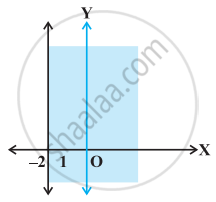# State whether the following statement is True or False. Graph of x > –2 is - Mathematics

MCQ
True or False

State whether the following statement is True or False.

Graph of x > –2 is• True

• False

#### Solution

This statement is False.

Explanation:

Because for x > –2, the line x = –2 has to be dotted i.e., the region does not include the points on the line x = –2.

Concept: Algebraic Solutions of Linear Inequalities in One Variable and Their Graphical Representation
Is there an error in this question or solution?
Chapter 6: Linear Inequalities - Solved Examples [Page 105]

#### APPEARS IN

NCERT Mathematics Exemplar Class 11
Chapter 6 Linear Inequalities
Solved Examples | Q 14 (vi) | Page 105
Share